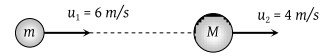A bomb of mass 30 kg at rest explodes into two pieces of masses 18 kg and 12 kg. The velocity of 18 kg mass is 6 ms–1. The kinetic energy of the other mass is

(1) 256 J

(2) 486 J

(3) 524 J

(4) 324 J

Concept Questions :-

Linear momentum
High Yielding Test Series + Question Bank - NEET 2020

Difficulty Level:

A shell initially at rest explodes into two pieces of equal mass, then the two pieces will

(1) Be at rest

(2) Move with different velocities in different directions

(3) Move with the same velocity in opposite directions

(4) Move with the same velocity in same direction

Concept Questions :-

Linear momentum
High Yielding Test Series + Question Bank - NEET 2020

Difficulty Level:

Two solid rubber balls A and B having masses 200 gm and 400 gm respectively are moving in opposite directions with velocity of A equal to 0.3 m/s. After collision the two balls come to rest, then the velocity of B is

(1) 0.15 m/sec

(2) 1.5 m/sec

(3) –0.15 m/sec

(4) None of the above

Concept Questions :-

Linear momentum
High Yielding Test Series + Question Bank - NEET 2020

Difficulty Level:

A cannon ball is fired with a velocity 200 m/sec at an angle of 60° with the horizontal. At the highest point of its flight ,it explodes into 3 equal fragments, one going vertically upwards with a velocity 100 m/sec, the second one falling vertically downwards with a velocity 100 m/sec. The third fragment will be moving with a velocity

(1) 100 m/s in the horizontal direction

(2) 300 m/s in the horizontal direction

(3) 300 m/s in a direction making an angle of 60° with the horizontal

(4) 200 m/s in a direction making an angle of 60° with the horizontal

Concept Questions :-

Linear momentum
High Yielding Test Series + Question Bank - NEET 2020

Difficulty Level:

A body of mass 5 kg explodes at rest into three fragments with masses in the ratio 1 : 1 : 3. The fragments with equal masses fly in mutually perpendicular directions with speeds of 21 m/s. The velocity of the heaviest fragment will be

(1) 11.5 m/s

(2) 14.0 m/s

(3) 7.0 m/s

(4) 9.89 m/s

Concept Questions :-

Linear momentum
High Yielding Test Series + Question Bank - NEET 2020

Difficulty Level:

A 238U nucleus decays by emitting an alpha particle of speed v ms–1. The recoil speed of the residual nucleus is (in ms–1

(1) –4v/234

(2) v/4

(3) –4v/238

(4) 4v/238

Concept Questions :-

Linear momentum
High Yielding Test Series + Question Bank - NEET 2020

Difficulty Level:

A smooth sphere of mass M moving with velocity u directly collides elastically with another sphere of mass m at rest. After collision their final velocities are V and v respectively. The value of v is

(1) $\frac{2uM}{m}$

(2) $\frac{2um}{M}$

(3) $\frac{2u}{1+\frac{m}{M}}$

(4) $\frac{2u}{1+\frac{M}{m}}$

Concept Questions :-

Linear momentum
High Yielding Test Series + Question Bank - NEET 2020

Difficulty Level:

A particle of mass m moving with horizontal speed 6 m/sec as shown in figure. If m << M then for one dimensional elastic collision, the speed of lighter particle after collision will be(1) 2m/sec in original direction

(2) 2 m/sec opposite to the original direction

(3) 4 m/sec opposite to the original direction

(4) 4 m/sec in original direction

Concept Questions :-

Linear momentum
High Yielding Test Series + Question Bank - NEET 2020

Difficulty Level:

A shell of mass m moving with velocity v suddenly breaks into 2 pieces. The part having mass m/4 remains stationary. The velocity of the other shell will be

(1) v

(2) 2v

(3) $\frac{3}{4}v$

(4) $\frac{4}{3}v$

Concept Questions :-

Linear momentum
High Yielding Test Series + Question Bank - NEET 2020

Difficulty Level:

Two equal masses m1 and m2 moving along the same straight line with velocities +3 m/s and –5 m/s respectively collide elastically. Their velocities after the collision will be respectively

(1) +4 m/s for both

(2) –3 m/s and +5 m/s

(3) –4 m/s and +4 m/s

(4) –5 m/s and +3 m/s

Concept Questions :-

Linear momentum